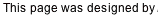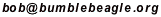## Du-Sum-Oh Solving Tutorial

Below is the puzzle we want to solve. We need to place the letters A-E in the cells so that each row contains exactly one of each letter. Ditto for each column and for each region. Several letters have been given to start us off.

This tutorial refers to rows and columns by number, and to regions by the starter letter in the region.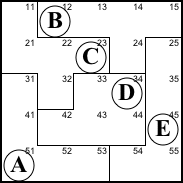Step 1. Row 4 and region D share the three purple cells below left. Thus the two blue cells that complete row 4 and the two pink cells that complete region D must contain the same two symbols. So cell 41 must be be D and cell 33 must be E. The result is shown below right.

41=D, 33=E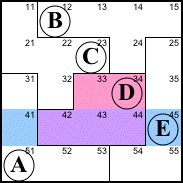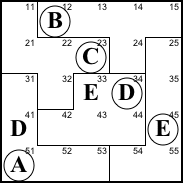Step 2. Similarly, column 1 and region C share the two purple cells below, so the blue cells (which complete column 1) must be the same as the pink cells (which complete region C).

This can be described as the little law of leftovers. Wherever a region overlaps a row or column, the parts outside the overlap (the leftovers) have to be the same.

31 must be C. The blue cells need A and D, but row 4 already has its D at 34. This forces the D to 22 and the A goes at 32.

31=C, 22=D, 32=A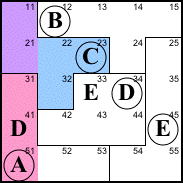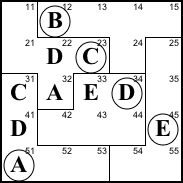Step 3. Row 3 only has one empty spot left, so we can finish it off.

35=B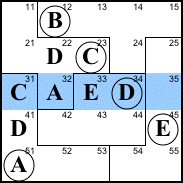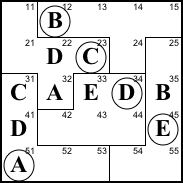Step 4. Column 1 lacks B and E. The B at 12 forces the B to 21 and 11 has to be E.

21=B, 11=E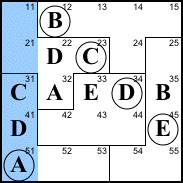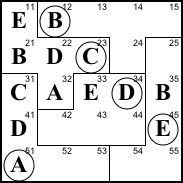Step 5. Equal leftovers means 24 is E. And then we can finish off row 2.

Although it doesn't come into play in this solution, single leftover situations like row 1 and region B can allow us to deduce that two cells are identical even without knowing either of the cells. In this puzzle, at first glance we can deduce that 11=24 and 54=15.

24=E, 25=A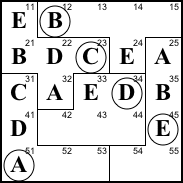Step 6. Column 2 lacks C and E. The E at 45 forces the E to 52, and the C goes to 42.

52=E, 42=C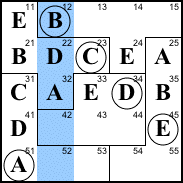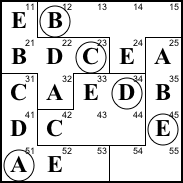Step 7. Equal leftovers means the pink cells must be C and D. The D at 34 forces the D to 55, and the C goes to 54. We can then finish off row 1 (53 is B) and column 5 (15 is C).

55=D, 54=C, 53=B, 15=C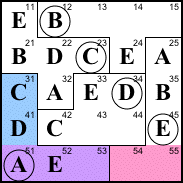Step 8. What's left easily falls into place. Row 1 lack A and D, and the D at 34 forces the D to 13. From there we finish off row 1 and column 3, and then column 4.

The solution is shown at right.

13=D, 14=A, 43=A, 44=B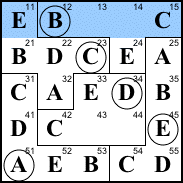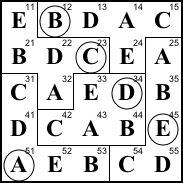Back to Bob’s main Du-Sum-Oh page.